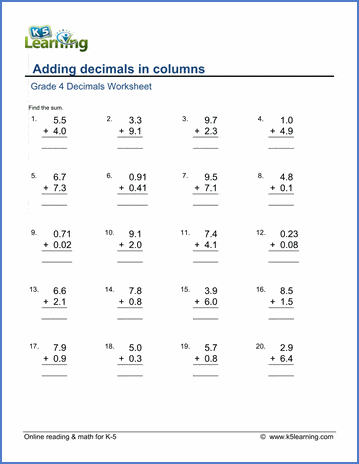# Decimals Worksheets

Our decimal worksheets start with the conversion of simple fractions (denominators of 10 or 100) to and from decimals and progress to operations with multi-digit decimals, rounding and repeating decimals.

Fractions to decimals worksheets

Comparing, ordering, adding and subtracting decimals

Fraction to / from decimals worksheets

Decimal multiplication worksheets

Decimal division worksheets

Fraction to / from decimals worksheets

Decimal multiplication worksheets

Decimal division worksheets

Topics include:

• Converting decimals to fractions and mixed numbers
• Converting fractions and mixed numbers to decimals (denominators of 10)
• Comparing and ordering decimals
• Subtract 1-digit decimals from whole numbers
• Subtract 1-digit decimals from whole numbers (missing minuend / subtrahend)
• Decimal subtraction (1 digit)

## Grade 4 fractions to decimals worksheets

• Convert decimals to fractions (tenths, hundredths)
• Convert decimals to mixed numbers (tenths, hundredths)
• Convert fractions to decimals (denominator of 10 or 100)
• Convert mixed numbers to decimals (denominator of 10 or 100)

• Comparing and ordering decimals
• Decimal addition (1 or 2 digits)
• Decimal addition in columns (2 digit)
• Decimal subtraction (1-digit)
• Decimal subtraction (1-digit), missing minuend/subtrehnds
• Decimal subtraction (1 or 2 digits)
• Decimal subtraction in columns (2 digit)
• Add / subtract decimals using money notation
• Word problems: adding and subtracting decimals

## Grade 5 fractions to / from decimals worksheets

• Convert decimals to fractions (tenths, hundredths), no simplification
• Convert decimals to fractions (tenths, hundredths), with simplification
• Convert decimals to mixed numbers
• Convert fractions to decimals (denominators of 10 or 100)
• Convert mixed numbers to decimals (denominators of 10 or 100)
• Convert mixed numbers to decimals (denominators of 10, 100 or 1000)
• Convert fractions to decimals (common denominators of 2, 4, 5, ...)
• Convert mixed numbers to decimals (common denominators of 2, 4, 5, ...)
• Convert fractions to decimals, with repeating decimals

• Addition with 0-1 decimal digits
• Addition with 2 decimal digits
• Addition with 3 decimal digits
• Subtract decimals (1-2 decimal digits)
• Subtract 1-2 digit decimals from whole numbers
• Missing minuend / subtrahend exercises
• Subtract decimals in columns
• Add / subtract with money notation

• Multiply whole numbers by 1 or 2 digit decimals
• Multiply decimals by decimals
• Missing factor exercises
• Multiplying by 10, 100, or 1,000 with up to 3 decimal digits
• Multiplying by 10, 100, or 1,000 with missing factors
• Multiply decimals by whole numbers in column form
• Multiply decimals by decimals in column form
• Multiply decimals with money notation.
• Decimal word problems (add / subtract / multiply)

## Grade 5 decimal division worksheets

• Dividing decimals (1-digit, 2-digit) by whole numbers
• Dividing decimals by whole numbers, missing dividends or divisors
• Dividing decimals by decimals, quotient a whole number
• Dividing whole numbers by 10, 100 or 1,000
• Dividing whole numbers by 10, 100 or 1,000, with missing dividends or divisors
• Dividing 1-digit decimals by 10, 100 or 1,000
• Long division of decimals by whole numbers, no rounding
• Division of decimals with money notation.

## Grade 6 fractions to / from decimals worksheets

• Convert decimals to decimals, with simplification
• Convert decimals to mixed numbers, with simplification
• Convert decimals to decimals (denominators are 10 or 100)
• Convert decimals to decimals (various denominators)
• Convert mixed numbers to decimals (various denominators)

• Adding decimals with 0-3 digits
• Adding decimals of varying length
• Subtracting decimals
• Subtracting decimals from whole numbers
• Missing minuend or subtrahend exercises
• Subtracting decimals in columns
• Subtracting decimals of varying length

## Grade 6 decimal multiplication worksheets

• Multiply whole numbers by decimals
• Multiply decimals by decimals mentally (1-3 decimal digits)
• Multiply decimals by 10, 100 or 1,000
• Multiply decimals by powers of ten (10000, 100000 etc)
• Multiply decimals in columns (up to 4 digits)
• Missing factor questions

## Grade 6 decimal division worksheets

• 1-2 digit decimals divided by whole numbers
• 1-2 digit decimals divided by decimals (quotient a whole number)
• Divide whole numbers by powers of ten
• Long division of decimals by whole numbers, division terminates
• Long division of decimals by whole numbers, with rounding
• Whole number long division, some answers repeat
• Long division of decimals by decimals

## Related topics

Fractions worksheets

Money worksheetsSample Decimals Worksheet

What is K5?

K5 Learning offers free worksheets, flashcards and inexpensive workbooks for kids in kindergarten to grade 5. Become a member to access additional content and skip ads.